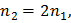# A point objectis kept at a distance of. The radius of curvature of the spherical surfaceis. The refractive index of the media areandwhich are as shown in diagram. Then,(1) If, image is virtual for all values of(2) Ifimage is virtual when(3) The image is real for all values ofand. Here, the correct statements is/are a) Only (2) b) Both (1) and (2) c) Only (1) d) (1), (2) and (3)

## Question ID - 152769 :- A point objectis kept at a distance of. The radius of curvature of the spherical surfaceis. The refractive index of the media areandwhich are as shown in diagram. Then,(1) If, image is virtual for all values of(2) Ifimage is virtual when(3) The image is real for all values ofand. Here, the correct statements is/are a) Only (2) b) Both (1) and (2) c) Only (1) d) (1), (2) and (3)

3537

Both (1) and (2)

Next Question :
 When sunlight is scattered by minute particles of atmosphere, the intensity of light scattered away is proportional to a)b)c)d)